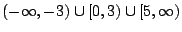Functions - Domain and Range; Composition

Sol 1 f is defined for all values of x (since f is a polynomial), so the domain of f is. Since the graph of f is a parabola which opens downward with vertex at, the set of y-coordinates for the points on the graph of f consists of all y-values with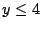; so the range of f is the interval.

Sol 2 f is defined whereor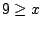, so the domain of f is the interval. Since,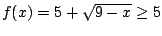for any x in the domain of f; so the range of f is contained in. If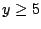,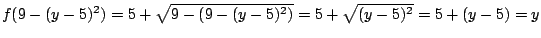(since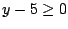), so the range of f is actually equal to.

Sol 3 f is defined for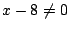or, so the domain of f is given by. To find the range of f, we must determine for which y-values the equationhas a solution for x. Multiplying both sides of this equation by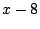givesor, so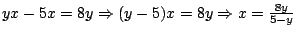. Therefore the equationhas a solution for x iffor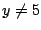, so the range of f is given by.

Sol 4 f is defined wherever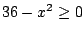(so the square root is defined) and(so the fraction is defined). Solving the inequalityorgives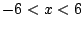, so the domain of f is.

Sol 5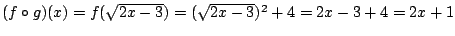, while.

Sol 6 We can letand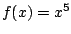, for example.

Sol 7 Since,and thereforeand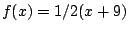.

Sol 8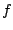is defined where, sogives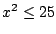. Taking the nonnegative square root of both sides gives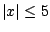or. Thereforeis the domain of.

Sol 9is defined where, so factoring gives the inequality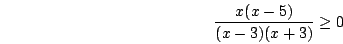.

Marking 0,5,3, and -3 on a number line and using the facts that all factors have odd exponents and that,

we get the following sign chart for:

Therefore the domain ofis given by